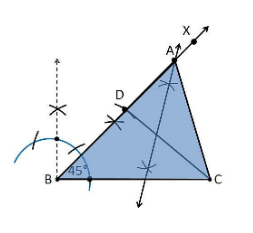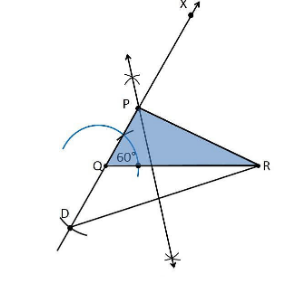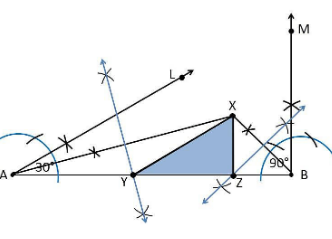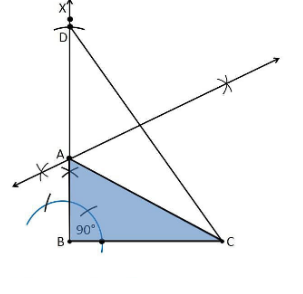# NCERT Solutions For Class 9 Math Chapter – 11 Exercise – 11.2

1. Construct a triangle ABC in which BC = 7cm, ∠B = 75° and AB+AC = 13 cm.

Construction Procedure:

The steps to draw the triangle of given measurement is as follows:

1. Draw a line segment of base BC = 7 cm

2. Measure and draw ∠B = 75° and draw the ray BX

3. Take a compass and measure AB+AC = 13 cm.

4. With B as the centre, draw an arc at the point be D

5. Join DC

6. Now draw the perpendicular bisector of the line DC and the intersection point is taken as A.

7.

Now join AC

8. Therefore, ABC is the required triangle.

2. Construct a triangle ABC in which BC = 8cm, ∠B = 45° and AB–AC = 3.5 cm.

Construction Procedure:

The steps to draw the triangle of given measurement is as follows:

1. Draw a line segment of base BC = 8 cm

2. Measure and draw ∠B = 45° and draw the ray BX

3. Take a compass and measure AB-AC = 3.5 cm.

4. With B as centre and draw an arc at the point be D on the ray BX

5. Join DC

6. Now draw the perpendicular bisector of the line CD and the intersection point is taken as A.

7. Now join AC

8. Therefore, ABC is the required triangle.3. Construct a triangle PQR in which QR = 6cm, ∠Q = 60° and PR–PQ = 2cm.

Construction Procedure:

The steps to draw the triangle of given measurement is as follows:

1. Draw a line segment of base QR = 6 cm

2. Measure and draw ∠Q = 60° and let the ray be QX

3. Take a compass and measure PR–PQ = 2cm.

4. Since PR–PQ is negative, QD will below the line QR.

5. With Q as centre and draw an arc at the point be D on the ray QX

6. Join DR

7. Now draw the perpendicular bisector of the line DR and the intersection point is taken as P.

8. Now join PR

9. Therefore, PQR is the required triangle.4. Construct a triangle XYZ in which ∠Y = 30°, ∠Z = 90° and XY+YZ+ZX = 11 cm.

Construction Procedure:

The steps to draw the triangle of given measurement is as follows:

1. Draw a line segment AB which is equal to XY+YZ+ZX = 11 cm.

2. Make an angle ∠Y = 30° from the point A and the angle be∠LAB

3. Make an angle ∠Z = 90° from the point B and the angle be ∠MAB

4. Bisect ∠LAB and ∠MAB at the point X.

5. Now take the perpendicular bisector of the line XA and XB and the intersection point be Y and Z, respectively.

6. Join XY and XZ

7. Therefore, XYZ is the required triangle5. Construct a right triangle whose base is 12cm and sum of its hypotenuse and other side is 18 cm.

Construction Procedure:

The steps to draw the triangle of given measurement is as follows:

1. Draw a line segment of base BC = 12 cm

2. Measure and draw ∠B = 90° and draw the ray BX

3. Take a compass and measure AB+AC = 18 cm.

4. With B as centre and draw an arc at the point be D on the ray BX

5. Join DC

6. Now draw the perpendicular bisector of the line CD and the intersection point is taken as A.

7. Now join AC

8. Therefore, ABC is the required triangle.